# Chapter 9 Linear Regression

### 1 变量间的关系

• 确定型关系vs不确定型关系
函数关系——一一对应的确定型关系设有两个变量x和y，变量y随变量x一起变化， 并完全依赖于x，当变量x取某个数值时，y依确定的关系取相应的值，则称y是x的函数，记为y=f(x)，其中x称为自变量，y称为因变量各观测点落在一条线上。
相关关系(correlation)——变量间关系不能用函数关系精确表达。一个变量的取值不能由另一个变量唯一确定。当变量x取某个值时， 变量y的取值可能有几个。各观测点分布在直线周围。https://www.autodeskresearch.com/publications/samestats

• 对变量之间关系密切程度的度量（只关心密切程度，无关因果关系）；
• 对两个变量之间线性相关程度的度量称为简单相关系数；
• 若相关系数是根据总体全部数据计算的，称为总体相关系数，记为ρ；
• 若是根据样本数据计算的，则称为样本相关系数，记为 r。

$$\rho=\frac{\sigma_{xy}}{\sigma_x\sigma_y}=\frac{E[(X-E(X))(Y-E(Y))]}{\sqrt{E(X-E(X))^2}\sqrt{E(Y-E(Y))^2}}$$

• 无量纲(Unitfree)；
• ρ的取值范围是 [-1,1]；
• |ρ|=1，为完全相关（ρ=1为完全正相关；ρ=-1为完全负相关）；
• ρ=0，不存在线性相关关系；
• -1≤ρ<0，为负相关，0<ρ≤1，为正相关；
• |ρ|越趋于1表示线性关系越密切；|ρ|越趋于0表示线性关系越不密切；
• 若X与Y相互独立，则ρ=0，但ρ=0，X与Y不一定相互独立；
• 若ρ= 0，且X与Y服从正态分布，则X与Y相互独立。

$$r=\frac{\sum(x_i-\bar x)(y_i-\bar y)}{\sqrt{\sum(x_i-\bar x)^2\cdot\sum(y_i-\bar y)^2}}或r=\frac{n\sum x_iy_i-\sum x_i\sum y_i}{\sqrt{n\sum x_i^2-(\sum x_i)^2}\cdot\sqrt{n\sum x_i^2-(\sum x_i)^2}}$$

• 无量纲(Unitfree)；
• r的取值范围是 [-1,1]；
• |r|=1，为完全相关（r=1为完全正相关；r=-1为完全负相关）；
• r=0，不存在线性相关关系；
• -1≤r<0为负相关，0<r≤1为正相关；
• |r|越趋于1表示线性关系越密切；|r|越趋于0表示线性关系越不密切；

r的抽样分布
r的抽样分布随总体相关系数和样本容量的大小而变化。当样本数据来自服从正态分布的总体时，随着n的增大，r的抽样分布趋于正态分布，尤其是在总体相关系数ρ很小或接近0时，趋于正态分布的趋势非常明显。而当ρ远离0时，除非n非常大，否则r的抽样分布呈现一定的偏态。当ρ为较大的正值时， r呈现左偏分布；当ρ为较小的负值时， r 呈现右偏分布。只有当ρ接近于0，而样本容量n很大时，才能认为r是接近于正态分布的随机变量。

（1） 提出假设：$H_0：\rho=0；H_1：\rho \neq0$
（2） 计算检验的统计量： $t=r\sqrt{\frac{n-2}{1-r^2}}\sim t(n-2)$
（3） 确定显著性水平α，并作出决策。

• 若$|t|>t_{α/2}$，拒绝$H_0$。
• 若$|t|<t_{α/2}$，不能拒绝$H_0$。

### 2 回归分析和简单线性回归分析

#### 2.2 简单线性回归分析

（1）简单线性回归模型的表示形式
$$y=\beta_0+\beta_1 x+\varepsilon$$
y是x的线性函数(部分)加上误差项(residual/random error term)。线性部分反映了由于x的变化而引起的y的变化。误差项ε是随机变量。反映了除x和y之间的线性关系之外的随机因素对y的影响，是不能由x和y之间的线性关系所解释的变异性。$β_0$和$β_1$称为模型的参数(interception, slope)。
（2）简单线性回归模型的基本假定

$$E(y)=\beta_0+\beta_1x$$

（3）简单线性回归方程(regression equation)

$$E(y)=\beta_0+\beta_1x$$

（4）估计的回归方程(estimated regression equation)

$$\hat y=b_0+b_1x$$

（5）最小二乘估计

$$argmin \sum_{i=1}^n(y_i-\hat y_i)^2=\sum_{i=1}^n(y_i-b_0-b_ix_i)^2$$

$$\begin{cases}b_1=\frac{n\sum_{i=1}^nx_iy_i-(\sum_{i=1}^nx_i)(\sum_{i=1}^ny_i)}{n\sum_{i=1}^nx_i^2-(\sum_{i=1}^nx_i)^2}\\b_0=\bar y-b_1\bar x\end{cases}$$

• 所有残差的和为0。所有残差的平方和最小；
• 回归直线经过变量X与Y的均值；
• 是$β_0和β_1$的无偏估计。

（7）回归直线的拟合优度

• 由于自变量 x 的取值不同造成的。
• 除 x 以外的其他因素(如x对y的非线性影响、测量误差等)的影响。
对一个具体的观测值来说， 变差的大小可以通过该实际观测值与其均值之差$y-\bar y$来表示。

$$\sum_{i=1}^n(y_i-\bar y)^2=\sum_{i=1}^n(\hat y_i-\bar y)^2+\sum_{i=1}^n(y_i-\hat y)^2$$

$$R^2=\frac{SSR}{SST}=\frac{\sum_{i=1}^n(\hat y_i-\bar y)^2}{\sum_{i=1}^n(y_i-\bar y)^2}=1-\frac{\sum_{i=1}^n(y_i-\hat y)^2}{\sum_{i=1}^n(\hat y_i-\bar y)^2}$$

• 反映回归直线的拟合程度；
• 取值范围在[0,1]之间；
• $R^2\rightarrow1$，说明回归方程拟合的越好；$R^2\rightarrow0$，说明回归方程拟合的越差；
• 对简单线性回归，判定系数等于相关系数的平方，r=(b1的符号)sqrt(R²)。

• 实际观察值与回归估计值离差平方和的均方根；
• 反映实际观察值在回归直线周围的分散状况；
• 对误差项ε的标准差σ的估计， 是在排除了x对y的线性影响后，y随机波动大小的一个估计量；
• 反映用估计的回归方程预测y时预测误差的大小。
计算公式为
$$s=\sqrt{\frac{\sum_{i=1}^n(y_i-\hat y_i)^2}{n-2}}=\sqrt{\frac{SSE}{n-2}}=\sqrt{MSE}$$

• 线性关系的显著性检验：检验自变量与因变量之间的线性关系是否显著，即检验x与y之间是否具有线性关系，或者说，检验自变量x对因变量y的影响是否显著；
• 回归系数的显著性检验：检验回归系数是否不等于0；
• 在简单线性回归中，线性关系的显著性检验等价于回归系数的显著性检验。
线性关系的检验
将回归均方(MSR)同残差均方(MSE)加以比较， 应用F检验来分析二者之间的差别是否显著。
回归均方：回归平方和SSR除以相应的自由度(自变量的个数p)；
残差均方：残差平方和SSE除以相应的自由度(n-p-1)。
• 提出假设：$H_0：\beta_1=0$ 线性关系不显著；
• 计算检验统计量F：
$$F=\frac{SSR/1}{SSE/(n-2)}=\frac{MSR}{MSE}\sim F(1,n-2)$$
• 确定显著性水平α，并根据分子自由度1和分母自由度n-2找出临界值$F_\alpha$。
• 作出决策：$若F>F_\alpha，拒绝H_0； 若F<F_\alpha，不拒绝H_0$。
回归系数的检验(检验步骤)
• 提出假设
$$H_0:\beta_1=0(没有线性关系)$$
$$H_1:\beta_1\neq 0(有线性关系)$$
• 计算检验的统计量
$$t=\frac{b_1}{s_{b_1}}\sim t(n-2)$$
• 确定显著性水平$\alpha$，并进行决策：
$$\left|t\right|\gt t_{\alpha/2}，拒绝H_0；\left|t\right|\lt t_{\alpha/2}，不拒绝H_0$$
显著性检验的几点注意
显著性关系的结论不意味着因果关系。显著性关系的结论也不能推出线性关系的结论，仅能说在x的样本观测之范围内，x和y是相关的，而且一个线性关系只揭示了y的变异的主要部分。当样本容量很大时，对于小的b1值也能得到统计上是显著的结果。

### 3 利用回归方程进行估计和预测

（1）点估计：y的平均值的点估计，y的个别值的点估计；
（2）区间估计：y的平均值的置信区间估计，y的个别值的预测区间估计。

（1）点估计

y的平均值的点估计

y的个别值的点估计

（2）区间估计

$$\hat y_0\pm t_{\alpha/2}(n-2)s\sqrt{\frac{1}{n}+\frac{(x_0-\bar x)^2}{\sum_{i=1}^n(x_i-\bar x)^2}}$$

x=均值时能得到y的平均值的最精确估计。

$$\hat y_0\pm t_{\alpha/2}(n-2)s\sqrt{1+\frac{1}{n}+\frac{(x_0-\bar x)^2}{\sum_{i=1}^n(x_i-\bar x)^2}}$$

• 置信水平(1-α)——区间宽度随置信水平的增大而增大；
• 数据的离散程度s——区间宽度随离散程度的增大而增大；
• 样本容量——区间宽度随样本容量的增大而减小；
• 用于预测的$x_p$与$\bar x$的差异程度，区间宽度随$x_p$与$\bar x$的差异程度的增大而增大。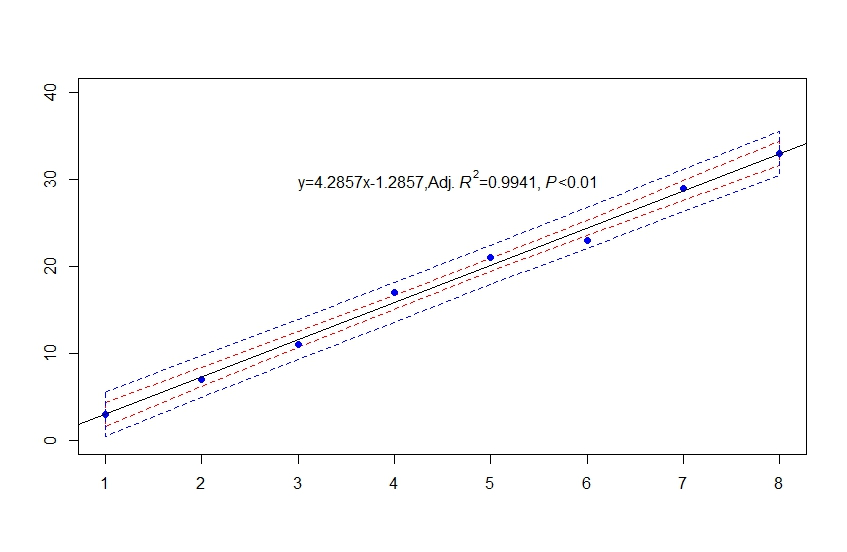### 4 残差分析

$$e_i=y_i-\hat y_i$$

• 检验线性的假设是否成立；
• 确定有关误差项ε的假定是否成立（正态分布；方差为常数；独立性）。
• 检测有影响的观测值。

• 表示残差的图形（关于x的残差图，关于y的残差图，标准化残差图）。
• 用直方图或正态概率图检验正态性。

• 残差除以它的标准差后得到的数值。 计算公式为
$$z_{e_i}=\frac{e_i}{s_{e_i}}=\frac{y_i-\hat y_i}{s_{e_i}}$$
• $e_i$是第i个残差的标准差， 其计算公式为
$$s_{e_i}=s_y\sqrt{1-h_i}=s_y\sqrt{1-(\frac{1}{n}+\frac{(x_i-\bar x)^2}{\sum(x_i-\bar x)^2})}$$

• 若假定成立， 标准化残差的分布也应服从正态分布。
• 在标准化残差图中， 大约有95%的标准化残差在-2到+2之间。

• 如果残差的标准差与因变量观测值的均值有线性关系，用log变换；
• 如果残差的方差与因变量观测值的均值有线性关系，用square root变换；
• 如果残差的标准差与因变量观测值的均值的平方有线性关系，用inverse变换；
• 如果残差的标准差与因变量观测值的均值的幂有线性关系，用power变换。

$$d=\frac{\sum_{t=2}^n(e_t-e_{t-1})^2}{\sum_{t=1}^ne_t^2}$$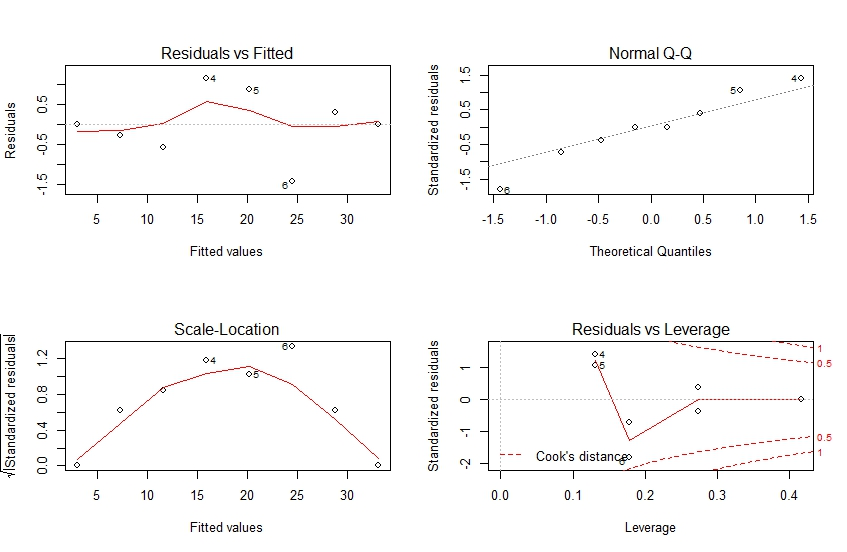• 如果异常值是一个错误的数据， 比如记录错误造成的， 应该修正该数据， 以便改善回归的效果；
• 如果是由于模型的假定不合理， 使得标准化残差偏大， 应该考虑采用其他形式的模型，比如非线性模型；
• 如果完全是由于随机因素而造成的异常值， 则应该保留该数据。

• 异常值也可以通过标准化残差来识别；
• 如果某一个观测值所对应的标准化残差较大， 就可以识别为异常值；
• 一般情况下，当一个观测值所对应的标准化残差小于-2或大于+2时，就可以将其视为异常值。

$$h_i=\frac{1}{n}+\frac{(x_i-\bar x)^2}{\sum(x_i-\bar x)^2}$$

### 5 多元线性回归(multiple regression model)

• 一个因变量与两个及两个以上自变量的回归。
• 描述因变量y如何依赖于自变量$x_1,x_2,\cdots,x_p$和误差项$\varepsilon$的方程，称为多元回归模型。
• 涉及 p 个自变量的多元回归模型可表示为
$$y=\beta_0+\beta_1x_1+\beta_2x_2+\cdots+\beta_px_p+\varepsilon$$
• $\beta_0,\beta_1,\beta_2,\cdots,\beta_p$是参数。
• $\varepsilon$是被称为误差项的随机变量。
• y是$x_1,x_2,\cdots,x_p$的线性函数加上误差项$\varepsilon$。
• $\varepsilon$包含在y里面但不能被p个自变量的线性关系所解释的变异性。

• 误差项ε是一个期望值为0的随机变量， 即E(ε)=0。
• 对于自变量$x_1,x_2,\cdots,x_p$的所有值，ε的方差$\sigma^2$都相同。
• 误差项ε是一个服从正态分布的随机变量，即$ε~N(0,\sigma^2)$，且相互独立。

$$E(y)=\beta_0+\beta_1x_1+\beta_2x_2+\cdots+\beta_px_p+\varepsilon$$

• $\beta_1,\beta_2,\cdots,\beta_p$称为偏回归系数。
• $\beta_i$表示假定其他变量不变，当$x_i$每变动一个单位时，y的平均变动值。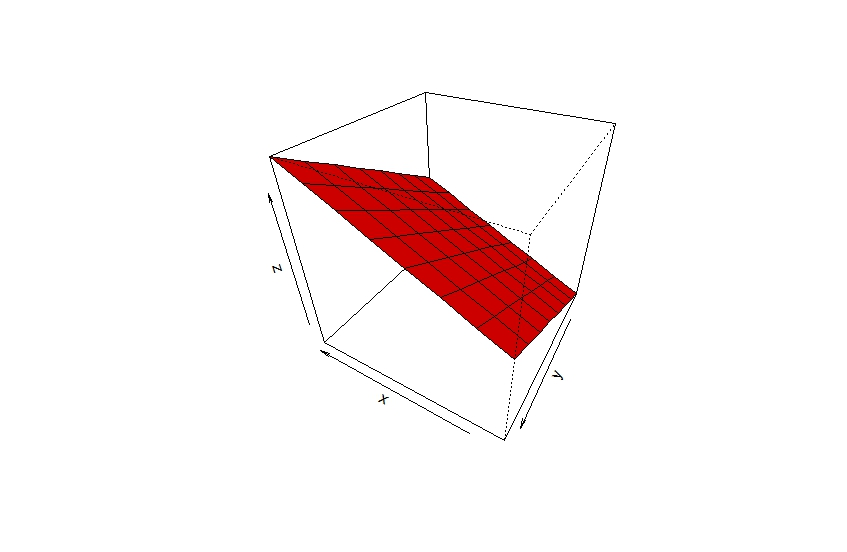$$\hat y=b_0+b_1x_1+b_2x_2+\cdots+b_px_p$$

$$argmin Q(b_0,b_1,b_2,\cdots,b_p)=\sum_{i=1}^n(y_i-\hat y_i)^2=\sum_{i=1}^ne_i^2$$

$$\begin{cases}\left. \frac{\partial Q}{\partial \beta_0} \right| _{\beta_0=b_0}=0\\\left. \frac{\partial Q}{\partial \beta_i} \right| _{\beta_i=b_i}=0(i=1,2,\cdots,p)\end{cases}$$

$$R^2=\frac{\sum_{i=1}^n(\hat y_i-\bar y)^2}{\sum_{i=1}^n(y_i-\bar y)^2}=\frac{SSR}{SST}=1-\frac{SSE}{SST}$$

$$R_a^2=1-(1-R^2)\times\frac{n-1}{n-p-1}$$

$$s=\sqrt{\frac{\sum_{i=1}^n(y_i-\hat y_i)^2}{n-p-1}}=\sqrt{\frac{SSE}{n-p-1}}=\sqrt{MSE}$$

• 如果是显著的， 因变量与自变量之间存在线性关系；
• 如果不显著， 因变量与自变量之间不存在线性关系。
（1）提出假设：
$H_0：\beta_1=\beta_2=\cdots=\beta_p=0$ 线性关系不显著；
$H_1：\beta_1,\beta_2,\cdots,\beta_p$ 至少有一个不等于0。
（2）计算检验统计量F：
$$F=\frac{SSR/p}{SSE/(n–p-1)}=\frac{MSR}{MSE}\sim F(p,n-p-1)$$
（3）确定显著性水平α，并根据分子自由度p和分母自由度n-p-1找出临界值$F_\alpha$。
（4）作出决策：$若F>F_\alpha，拒绝H_0$。

• 线性关系检验通过后，对各个回归系数进行检验。
• 对每一个自变量单独应用 t 检验统计量进行检验。
（1）提出假设
$$H_0:\beta_i=0(自变量x_i与因变量y没有线性关系)$$
$$H_1:\beta_i\neq 0(自变量x_i与因变量y有线性关系)$$
（2）计算检验的统计量
$$t=\frac{b_i}{s_{b_i}}\sim t(n-p-1)，s_{b_i}=\frac{s}{\sqrt{\sum(x_i-\bar x)^2}}$$
（3）确定显著性水平$\alpha$，并进行决策：
$$\left|t\right|\gt t_{\alpha/2}，拒绝H_0；\left|t\right|\lt t_{\alpha/2}，不拒绝H_0$$

$$b_i\pm t_{\alpha/2}(n-p-1)s_{b_i}$$

$$s_{b_i}=\frac{s}{\sqrt{\sum(x_i-\bar x)^2}}$$

### 6 多重共线性(multicollinearity)

• 检测多重共线性的最简单的一种办法是计算模型中各对自变量之间的相关系数， 并对各相关系数进行显著性检验；
若有一个或多个相关系数显著， 就表示模型中所用的自变量之间相关，存在着多重共线性。
• 如果出现下列情况，暗示存在多重共线性：
模型中各对自变量之间显著相关。
当模型的线性关系检验(F检验)显著时，几乎所有回归系数的t检验却不显著。
回归系数的正负号与预期的相反。

VIF (variance inflation factor) 用以测量如果自变量相关对估计的回归系数的变异程度的影响。
$VIF_j$的定义:
$$VIF_j=\frac{1}{1-R_j^2}$$
$R_2^j$是第j个自变量对其它自变量进行回归的判定系数。VIF=1表示所对应自变量与其它自变量无线性关系。VIF值越大，多重共线性越严重。如果$VIF_j$>5，$x_j$与其它自变量高度相关。

### 8 非线性回归

$$y_i=\beta_0+\beta_1x_i+\beta_2x_i^2+\varepsilon$$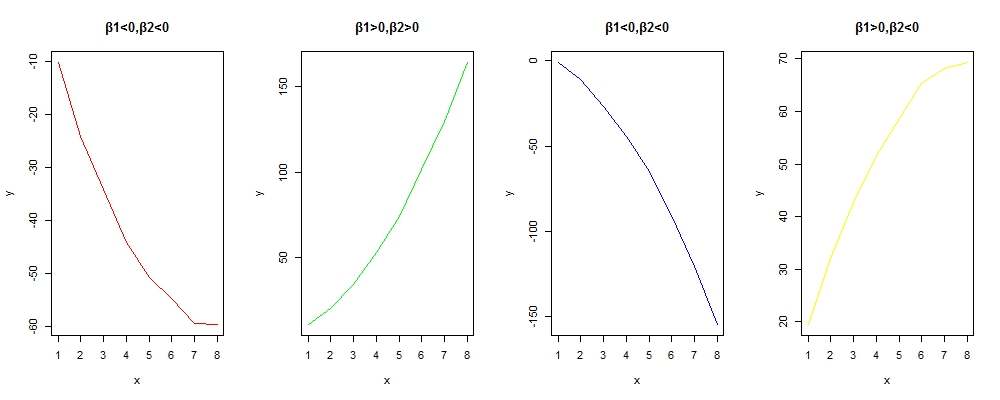• 总体显著性检验
$$F test statistic=\frac{MSR}{MSE}$$
• 二阶检验
比较二阶模型
$$y=\beta_0+\beta_1x_1+\beta_2x^2+\varepsilon$$
线性模型
$$y=\beta_0+\beta_1x_1+\varepsilon$$
假设：
$H_0:β_2=0 (没有二阶项)$
$H_1:β_2\neq 0 (需要二阶项)$

（2）交互作用

$$y=\beta_0+\beta_1x_1+\beta_2x_2+\beta_3x_1x_2+\varepsilon$$

• 交互作用模型:
$$y=\beta_0+\beta_1x_1+\beta_2x_2+\beta_3x_1x_2+\varepsilon$$
• 假设：
$$H_0：\beta_3=0 （x_1和x_2无交互作用）$$
$$H_1：\beta_3\neq 0 （x_1和x_2有交互作用）$$

（3）其他非线性回归

• 双曲线
基本形式：$y=\frac{x}{\alpha x+\beta}$
线性化方法：$令y’=\frac{1}{y}，x’=\frac{1}{x}，则有y’=\alpha+\beta x’$• 幂函数曲线
基本形式：$y=\alpha x^\beta$
线性化方法：$两端取对数得：lgy=lg\alpha+\beta lgx，y’=lgy，x’=lgx，则有y’=lg\alpha+\beta x’$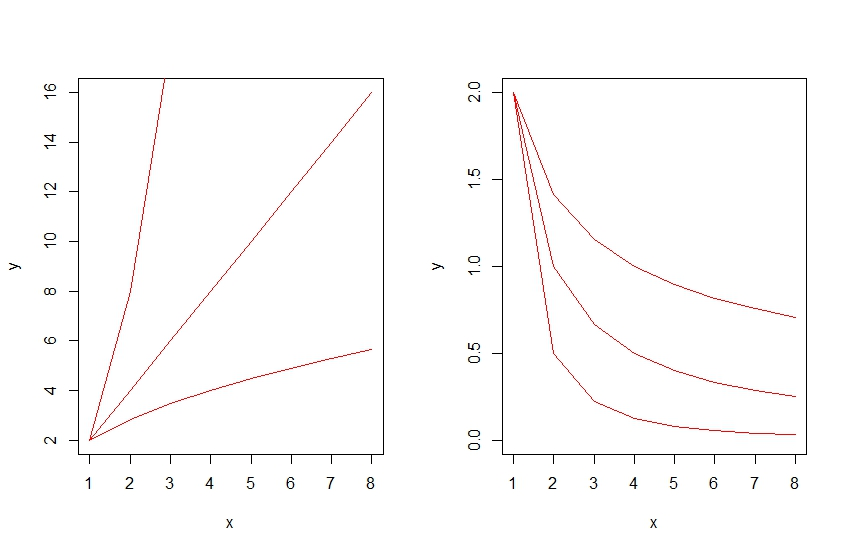• 对数曲线
基本形式：$y=\alpha+\beta lnx$
线性化方法：$x’=lnx，则有y’=\alpha+\beta x’$• 指数曲线
基本形式：$y=\alpha^{\beta x}$
线性化方法：$两端取对数得：lny=ln\alpha+\beta x，y’=lny，则有y’=ln\alpha+\beta x$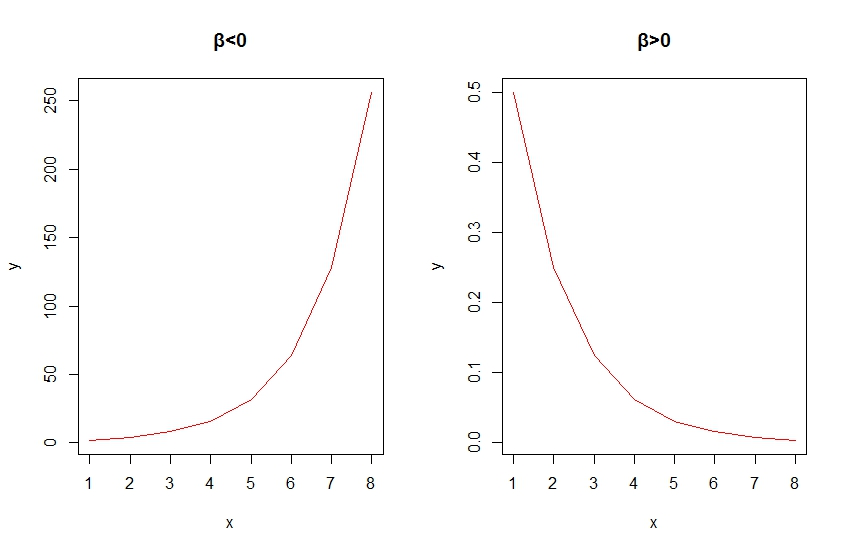• S型曲线
基本形式：$y=\frac{1}{\alpha+\beta e^{-x}}$
线性化方法：$令y’=1/y，x’=e^{-x}，则有y’=\alpha+\beta x’$### 9 建立回归模型

• 俭约的模型–用尽可能少的变量来提供足够精度的预测。
• 将不重要的变量除去更容易对模型进行解释。
• 发生多重共线性的可能变小。

$$F=\frac{SSE(x_1,x_2,\cdots,x_p)-SSE(x_1,x_2,\cdots,x_q,x_{q+1},\cdots,x_p)}{\frac{SSE(x_1,x_2,\cdots,x_q,x_{q+1},\cdots,x_p)}{n-p-1}}$$
$$F\sim F(p-q,n-p-1)$$
F越大，说明增加变量减少预测误差的效果越显著。

• 向前选择(Forward Selection)
1. 从没有自变量的模型开始。
2. 如果所有的F统计量的p-值大于预先设定的终止值，说明增加任一变量效果不显著，停止。
3. 否则，加入具有最大F统计量值的变量。
4. 重新回归， Go to Step 2。
• 后向消元(Backward Elimination)
1. 从包含所有自变量的模型开始。
2. 如果所有的F统计量的p-值小于预先设定的终止值，说明减少任一变量效果显著，停止。
3. 否则，删除具有最小F统计量值的变量。
4. 重新回归， Go to Step 2。
• 逐步回归(Stepwise regression procedure)
向前选择和后向消元的结合。
1.先检查是否有变量需从模型中删除。
2.再检查增加一个变量是否能改善模型。
3.重复以上过程。
注意： α进≤α出，否则F进<F<F出，会导致无限循环。
• 最佳子集回归(Best-subset approach)
对所有可能的自变量组合进行估计。找出具有最大的修正判定系数$adj.R^2$和最小的估计误差标准差$s_ε$。

### 10 回归中的常见错误

（1）没有检验线性关系假设

（2）只看结果不看图表

（3）用回归系数判定变量的重要性

（4）没有确定置信区间

（5）没有计算判定系数

（6）错误解释相关系数

$R^2$给出变异由回归解释的百分比，不是R。

（7）使用强相关的自变量

（8）用回归模型预测观测值范围之外的区域

（9）观测值取值范围太小

(10)包括太多的自变量

（11）认为好的预测变量是好的控制变量

（12）线性回归结果会给人以误导

### 11 Logistic 回归

Logistic回归提出的目的是为了解决二值化数据的回归问题。那么为什么简单线性回归模型不适合二值化数据的回归呢？详细原因可见如下图。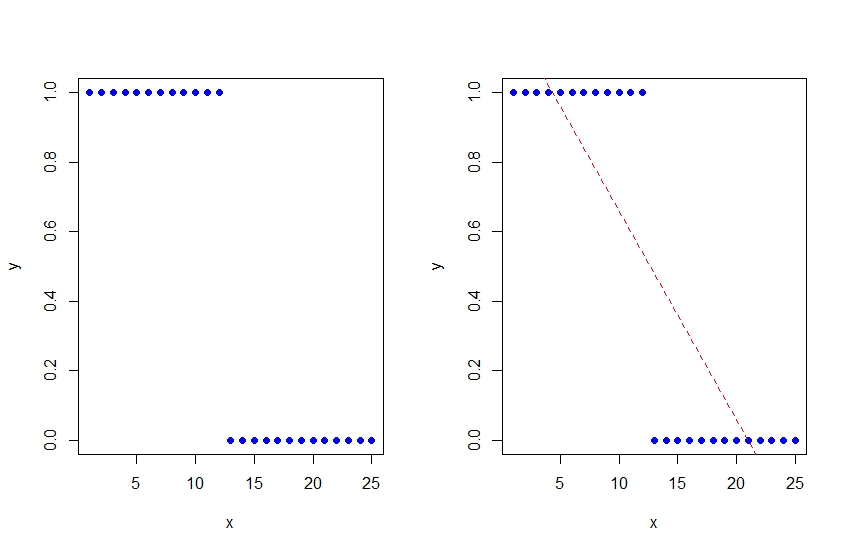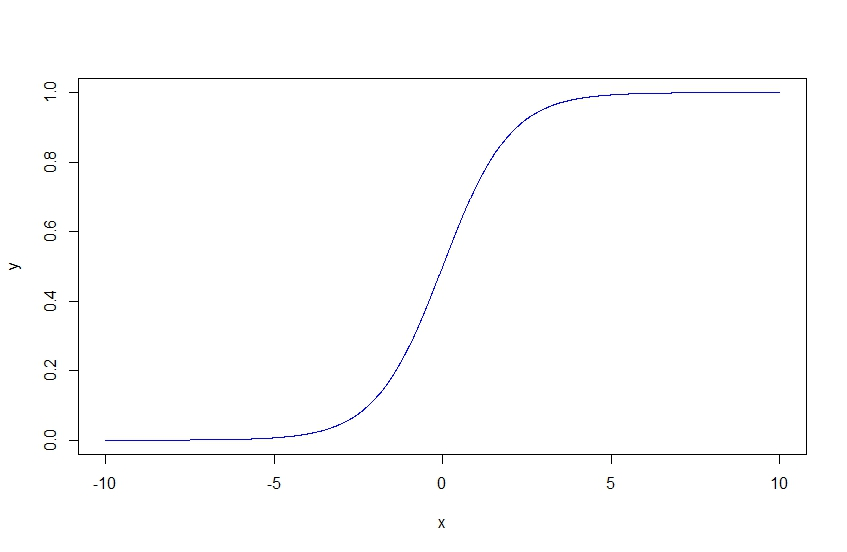Logistic的基本形式为
$$\pi_i’=ln(\frac{\pi_i}{1-\pi_i})=\beta_0+\beta_1x_i$$

• 都有一个二值化（或分类）变量：
• 都涉及到预测的思想机会，概率，比例或百分比。
• 不像其他的预测情况，y值是有界的。

Logistic 回归与简单线性回归

logistic回归是一种统计技术，可以用二值化变量问题中。回归虽有相似之处，但它不同于普通最小二乘法。识别重要和相似之处是两种技术的区别。